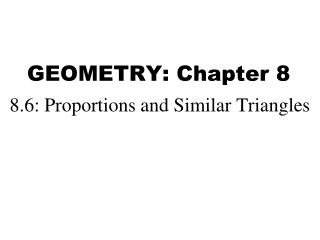DownloadDownload PresentationGEOMETRY: Chapter 8

# GEOMETRY: Chapter 8

Télécharger la présentation## GEOMETRY: Chapter 8

- - - - - - - - - - - - - - - - - - - - - - - - - - - E N D - - - - - - - - - - - - - - - - - - - - - - - - - - -
##### Presentation Transcript

1. GEOMETRY: Chapter 8 8.6: Proportions and Similar Triangles

2. Theorem 8.4. Triangle Proportionality Theorem If a line parallel to one side of a triangle intersects the other two sides, then it divides the two side proportionally. Image taken from: Geometry. McDougal Littell: Boston, 2007. P. 397.

3. Theorem 8.5.Converse of the Triangle Proportionality Theorem If a line divides two sides of a triangle proportionally, then it is parallel to the third side. Image taken from: Geometry. McDougal Littell: Boston, 2007. P. 397.

4. Ex. 1: Image taken from: Geometry. McDougal Littell: Boston, 2007. P. 398.

5. Ex. 1: Answer: 9 Image taken from: Geometry. McDougal Littell: Boston, 2007. P. 398.

6. Ex. 2: A brace is added to a tree house as shown. Explain why the brace is not parallel to the floor. Image taken from: Geometry. McDougal Littell: Boston, 2007. P. 389.

7. Ex. 2: A brace is added to a tree house as shown. Explain why the brace is not parallel to the floor. Image taken from: Geometry. McDougal Littell: Boston, 2007. P. 389.

8. Theorem 8.6: If three parallel lines intersect two transversals, then they divide the transversals proportionally. Image taken from: Geometry. McDougal Littell: Boston, 2007. P. 398.

9. Theorem 8.7: If a ray bisects an angle of a triangle, then it divides the opposite side into segments whose lengths are proportional to the lengths of the other two sides. Image taken from: Geometry. McDougal Littell: Boston, 2007. P. 398.

10. Ex. 3. Using the information in the diagram, find the distance between First Street and Main Street. Image taken from: Geometry. McDougal Littell: Boston, 2007. P. 399.

11. Ex. 3. Using the information in the diagram, find the distance between First Street and Main Street. Answer: 510 m Image taken from: Geometry. McDougal Littell: Boston, 2007. P. 399.

12. Ex.4 Image taken from: Geometry. McDougal Littell: Boston, 2007. P. 391.

13. Ex.4 Answer: 22 6/7 Image taken from: Geometry. McDougal Littell: Boston, 2007. P. 391.

14. 8.6, p. 502, #3-14 all, 21-28 all (20 questions)